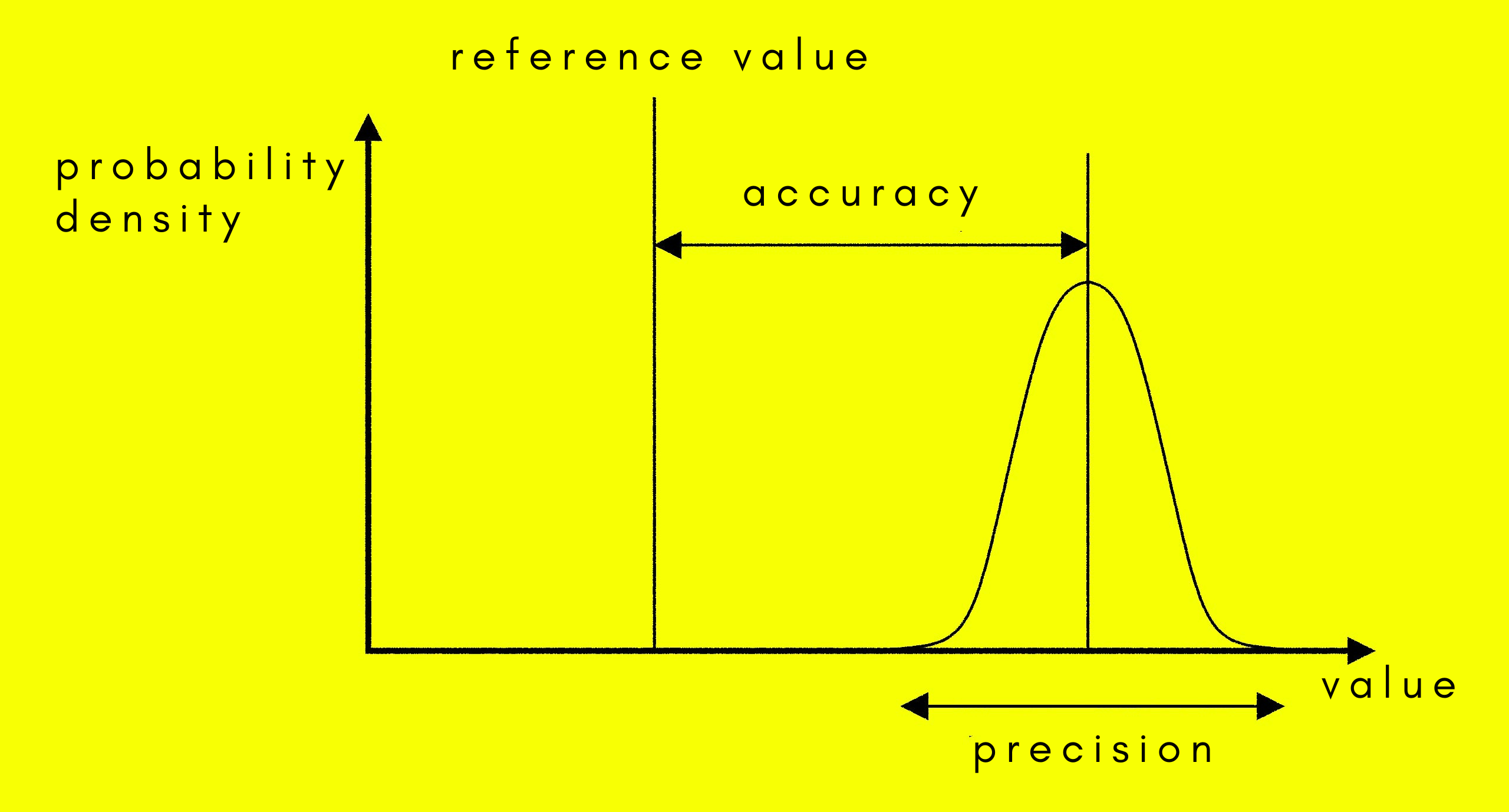AaccuracyThe difference between accuracy and precision.

Accuracy may refer to any of the following:

1. A qualitative assessment of correctness or freedom from error.

2. A quantitative measure of the magnitude of error. It differs from precision, in that it refers to how close measurements are to a specific value, whereas precision is refers to how close measurements to each other.

3. The measure of an instrument's capability to approach a true or absolute value. It is a function of precision and bias.

In science and engineering, the accuracy of a system of measurement is the degree of closeness of measurements of a quantity to that quantity's true value. The precision of a measurement system, on the other hand, is the degree to which repeated measurements under the same conditions give the same results. Colloquially, the two terms "accuracy" and "precision" are often used synonymously, but in science there is a clear distinction. In statistics, the terms "bias" and "variability" are often instead of accuracy and precision: Bias is the amount of inaccuracy and variability the amount of imprecision.

A measurement system can be accurate but not precise, precise but not accurate, neither, or both. For example, if an experiment contains a systematic error, then increasing the sample size generally increases the precision but doesn't improve accuracy. The result would be a consistent yet inaccurate string of results from the flawed experiment. Eliminating the systematic error improves accuracy but doesn't change precision. A measurement system is considered valid if it is both accurate and precise. Related terms include bias (non-random or directed effects caused by a factor or factors unrelated to the independent variable) and error (random variability). See also calibration.